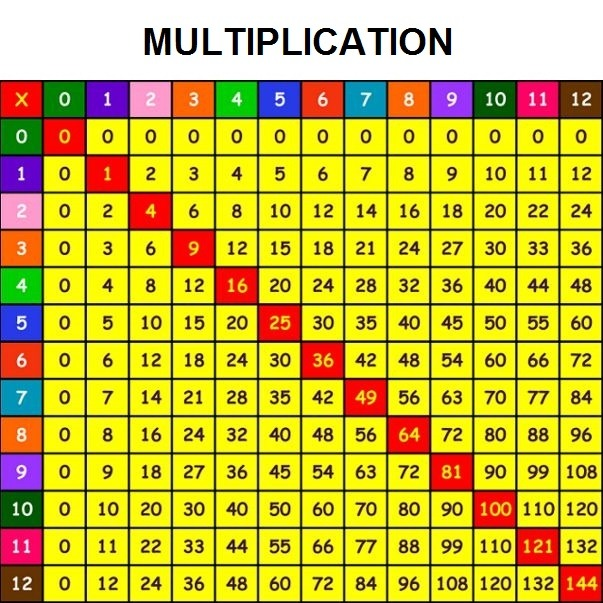Basic math operations - Addition, subtraction, multiplication and division

# Basic math operations

Basic math operations include four basic operations:
Subtraction (-)
Multiplication (* or x) and
Division ( : or /)

These operations are commonly called arithmetic operations. Arithmetic is the oldest and most elementary branch of mathematics.

In this and other related lessons, we will briefly explain basic math operations. Keep in mind, even though the operations and the examples shown here are pretty simple, they provide the basis for even the most complex operations used in mathematics.

Addition is a mathematical operation that explains the total amount of objects when they are put together in a collection.

For example, let’s say that Jimmy has 2 apples and Laura has 3 apples, and that we want to find out how many apples they have together. By adding them together, we see that both of them combined have 5 apples (2 Jimmy’s apples + 3 Laura’s apples = 5 apples in total). As you can see, the addition is signified by the “plus sign (+)”.Addition can also be used to perform operations with negative numbers, fractions, decimal numbers, functions, etc. There are several arithmetic properties that are typical for addition:
1. Commutative property
2. Associative property
3. Identity property

## Subtraction

Subtraction is the arithmetic operation that is the opposite of addition. Subtraction is used when you want to know how many objects are left in the group after you take away a certain amount of objects from that group.

For example, Maggie has 5 apples. She gives 2 apples to her friend, Paul. How many apples does she have? She has 3 apples (5 apples that she had – 2 apples that she gave to Paul = 3 apples that are left to her).As you can see, subtraction is determined by the “minus (-) symbol”. Subtraction can also be used to perform operations with negative numbers, fractions, decimal numbers, functions, etc.

## Multiplication

Multiplication is the third basic math operation. When you multiply two numbers, this is the same as adding the number to itself as many times as the value of the other number is. Think of it like this: You have 5 groups of apples and each group has 3 apples. One of the ways you can find out how many apples you have is this one:

3 apples + 3 apples + 3 apples + 3 apples + 3 apples = 15 apples in total

You can see that it is way too much work (especially if you have larger numbers), so you can use multiplication to solve this problem:

5 group of apples x 3 apples in every group = 15 apples in total

This could be even easier by using the table of multiplication.Multiplication is signified with multiplication sign “x”, and it is often read as “times” or “multiplied by”. So if you had an expression like “3 x 4”, you could read it as “3 times 4” or “3 multiplied by 4”. In the other words, the expression of multiplication signifies the number of times one number is multiplied by another number.

$\ 3 * 4 = 12$  The number 3 is multiplied in this equation 4 times, and when you multiply 3 by 4 you get the number 12 as a result.

## Division

Division is the fourth basic math operation. Basically, you can say that dividing means splitting objects into equal parts or groups.

For example, you have 12 apples that need to be shared equally between 4 people. So, how many apples will each person get? Each person will get 3 apples (12 apples / 4 people = 3 apples per person). The division is the opposite of multiplication:

$\ 3 * 4 = 12$

$\ 4 * 3= 12$

$\frac{12}{4} = 3$

$\frac{12}{3}=4$

For easier understanding of the division of one number by another, use the table of division:## Basic math operations

The basic math operations are addition, subtraction, multiplication, and division. Depending on the directions for the math problem, you may see different words:

• Subtraction: subtracting, subtract
• Multiplication: multiplying, multiply
• Division: dividing, divide

In mathematics when you perform computational actions, you must have in mind that there is a sequence that need to be respected in order to do calculations properly.

Addition and subtraction are first degree mathematical operations. Multiplication and division are second degree mathematical operations. This means:

● If same degree operations, we resolve them by their order (from left to right).

For example:
$\ 18 – 2 + 4 = 16 + 4 = 20$  This only applies if there are no brackets in the equation. If there are brackets, we firstly resolve numbers in the brackets.

$\ 18 – ( 2 + 4 ) = 18 – 6 = 12$  Notice the difference in results, even with same numbers.

● If there are different degree operations, we resolve it by the degree order – multiplication and division first and addition and subtraction after.

For example:

$\ 2 + 3 * 4 = 2 + 12 = 14$

Numbers in brackets are need to be resolve firstly in any case!